##### Trigonometry Workbook For DummiesQuadratic equations are nice to work with because, when they don’t factor, you can solve them by using the quadratic formula. The types of quadratic trig equations that you can factor are those like tan2 x = tan x, 4cos2 x – 3 = 0, 2sin2 x + 5sin x – 3 = 0, and csc2 x + csc x – 2 = 0. Notice that they all have the telltale trigonometry function raised to the second degree. The following examples show you how to handle them.

The first two examples have just two terms. One has two variable terms, and the other has just one variable term. In the first example, you put both terms on the left and then factor out the variable or trig term.

Solve tan2 x = tan x for the values of x such that 0 x < 2.

1. Move the term on the right to the left by subtracting it from both sides.

tan2 x – tan x = 0

Don’t divide through by tan x. You’ll lose solutions.

2. Factor out tan x.

tan x (tan x – 1) = 0

3. Set each of the two factors equal to 0.

tan x = 0 or tan x – 1 = 0

4. Solve for the values of x that satisfy both equations.

If tan x = 0, then x = tan–1(0) = 0, .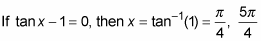In this next example, the binomial doesn’t factor easily as the difference of two squares, because the 3 isn’t a perfect square, and you have to use a radical in the factorization. A nice, efficient way to solve this equation is to move the 3 to the right and take the square root of each side.

Solve for all the possible solutions of 4cos2 x – 3 = 0 in degrees.

1. Move the number to the right by adding 3 to each side.

4cos2 x = 3

2. Divide each side by 4, and then take the square root of each side to solve for cos x.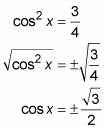3. Solve the two equations for the values of x.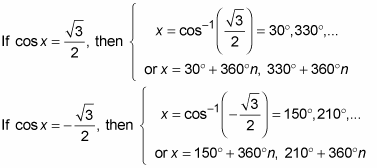When you consider all the multiples of 360 degrees added to the four base angles, you find that this equation has lots and lots of solutions.

The next two examples involve using un-FOIL — a technique for determining the product of which two binomials gives you a particular quadratic trinomial. Sometimes, when the pattern in the trinomial is obscured, you may want to first substitute some other variable for the trig function to help figure out how you factor it. In this example, you will do this to solve 2sin2 x + 5sin x – 3 = 0 for all the values of x between 0 and 360 degrees.

1. Replace each sin x with y.

2y2 + 5y – 3 = 0

2. Factor the trinomial as the product of two binomials.

(2y – 1)(y + 3) = 0

3. Replace each y with sin x.

(2sin x – 1)(sin x + 3) = 0

4. Set each factor equal to 0.

2sin x – 1 = 0 or sin x + 3 = 0

5. Solve the two equations for the values of x that satisfy them.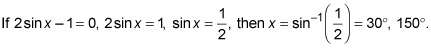If sin x + 3 = 0, sin x = –3, then x = sin–1(–3). This result is nonsense, because the sine function only produces values between –1 and 1 — so this factor doesn’t produce any solutions.

The only two solutions are 30 and 150 degrees.

This next example factors fairly easily, but it involves a reciprocal function. Solve csc2 x + csc x – 2 = 0 for any angles between 0 and 2p radians.

1. Factor the quadratic trinomial into the product of two binomials.

(csc x + 2)(csc x – 1) = 0

2. Set each factor equal to 0.

csc x + 2 = 0 or csc x – 1 = 0

3. Solve the two equations for the values of x that satisfy them.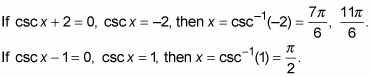An alternate way of dealing with these two binomial equations is to change them by using the reciprocal identity and writing the reciprocal of the number. For the first equation, you’d change from cosecant to sine: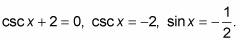Do the same for the second equation: csc x – 1 = 0, csc x = 1, sin x = 1. You’d then solve the inverse equations.